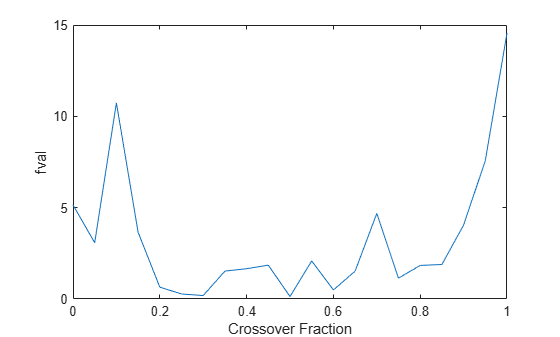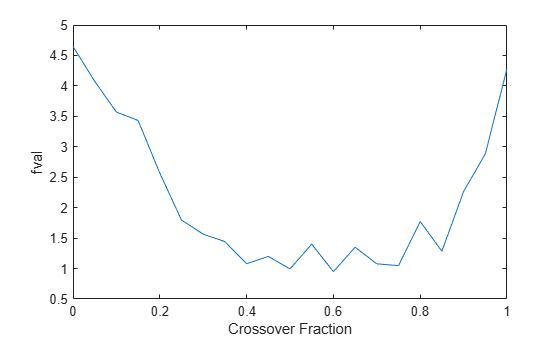# Run `ga` from a File

The command-line interface enables you to run the genetic algorithm many times, with different options settings, using a file. For example, you can run the genetic algorithm with different settings for Crossover fraction to see which one gives the best results. The following code runs the function `ga` 21 times, varying `options.CrossoverFraction` from 0 to 1 in increments of 0.05, and records the results.

```options = optimoptions('ga','MaxGenerations',300,'Display','none'); rng default % for reproducibility record=[]; for n=0:.05:1 options = optimoptions(options,'CrossoverFraction',n); [x,fval]=ga(@rastriginsfcn,2,[],[],[],[],[],[],[],options); record = [record; fval]; end```

Plot the values of `fval` against the crossover fraction.

```plot(0:.05:1, record); xlabel('Crossover Fraction'); ylabel('fval')```The plot suggests that you get the best results by setting `options.CrossoverFraction` to a value somewhere between 0.4 and 0.8.

You can get a smoother plot of `fval` as a function of the crossover fraction by running `ga` 20 times and averaging the values of fval for each crossover fraction.

```options = optimoptions('ga','MaxGenerations',300,'Display','none'); rng default % for reproducibility record=[]; fval = zeros(20,1); for n=0:.05:1 options = optimoptions(options,'CrossoverFraction', n); for i = 1:20 [x,fval(i)]=ga(@rastriginsfcn,2,[],[],[],[],[],[],[],options); end meanf = mean(fval); record = [record; meanf]; end plot(0:.05:1, record); xlabel('Crossover Fraction'); ylabel('fval')```This plot also suggests the range of best choices for `options.CrossoverFraction` is 0.4 to 0.8.